Test: Power Electronics- 4

# Test: Power Electronics- 4

Test Description

## 10 Questions MCQ Test GATE Electrical Engineering (EE) 2023 Mock Test Series | Test: Power Electronics- 4

Test: Power Electronics- 4 for Electrical Engineering (EE) 2023 is part of GATE Electrical Engineering (EE) 2023 Mock Test Series preparation. The Test: Power Electronics- 4 questions and answers have been prepared according to the Electrical Engineering (EE) exam syllabus.The Test: Power Electronics- 4 MCQs are made for Electrical Engineering (EE) 2023 Exam. Find important definitions, questions, notes, meanings, examples, exercises, MCQs and online tests for Test: Power Electronics- 4 below.
Solutions of Test: Power Electronics- 4 questions in English are available as part of our GATE Electrical Engineering (EE) 2023 Mock Test Series for Electrical Engineering (EE) & Test: Power Electronics- 4 solutions in Hindi for GATE Electrical Engineering (EE) 2023 Mock Test Series course. Download more important topics, notes, lectures and mock test series for Electrical Engineering (EE) Exam by signing up for free. Attempt Test: Power Electronics- 4 | 10 questions in 30 minutes | Mock test for Electrical Engineering (EE) preparation | Free important questions MCQ to study GATE Electrical Engineering (EE) 2023 Mock Test Series for Electrical Engineering (EE) Exam | Download free PDF with solutions
 1 Crore+ students have signed up on EduRev. Have you?
Test: Power Electronics- 4 - Question 1

### Which of the following are the advantages of freewheeling of load current in phase – controlled converters? 1. It reduces the reactive power demand of the converter 2. It improves the displacement factor 3. It reduces the generated ripple voltage on the DC side of an SCR phase controlled converter 4. It improves the input PF. Select the correct answer using the codes given below

Detailed Solution for Test: Power Electronics- 4 - Question 1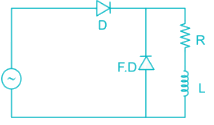1. It prevents the output voltage from becoming negative

2. System efficiency is improved because energy stored in L is transferred to load R through FD.

3. Load current waveform is more smooth.

4. It reduces the reactive power demand of the converter.

5. It improves the displacement factor.

6. It improves distortion factor.

7. It improves the input PF.

*Answer can only contain numeric values
Test: Power Electronics- 4 - Question 2

### A battery is charged by a single phase half wave diode rectifier. The supply voltage is 220 V (rms), 50 Hz and the battery emf is constant at 80 V. then PIV rating of the diode should be___ (in V)

Detailed Solution for Test: Power Electronics- 4 - Question 2

PIV = Vm + E = 220√2 + 80 = 3 91.13 V

Test: Power Electronics- 4 - Question 3

### A single phase full – wave half controlled bridge converter feeds an inductive load. The two SCRs in the converter are connected to a common DC bus. The converter has to have a freewheeling diode.

Detailed Solution for Test: Power Electronics- 4 - Question 3

Single phase full wave half controlled bridge converter feeds an Inductive load. The two SCRs in the converter are connected to a common dc bus. The converter has to have freewheeling diode because the converter does not provide for freewheeling for high value of triggering angles

Test: Power Electronics- 4 - Question 4

If the commutation angle of a diode rectifier (due to source inductive effect) is μ, then the inductive voltage regulation will be

Detailed Solution for Test: Power Electronics- 4 - Question 4

Voltage regulation =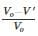for single phase full converter bridge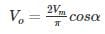due to source inductance effect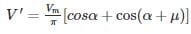Where μ is overlapping angle and α is firing angle
for α = 0, V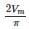and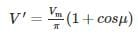Voltage regulation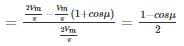Test: Power Electronics- 4 - Question 5

A single phase controlled rectifier bridge consists of one SCR and three diode as shown in figure find the waveform of current through T1.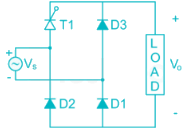Detailed Solution for Test: Power Electronics- 4 - Question 5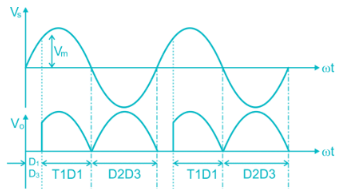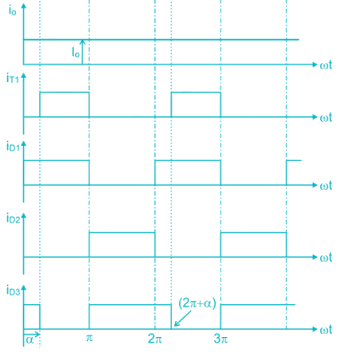*Answer can only contain numeric values
Test: Power Electronics- 4 - Question 6

1 ϕ full bridge converter with freewheeling diode feeds an inductive load. Load resistance is 15 Ω and it has a large inductance providing constant and ripple free dc current input to converter from ideal 220 V, 50 Hz single phase source. For a firing angle of 30°, the value of output current is _________A.

Detailed Solution for Test: Power Electronics- 4 - Question 6

the average output voltage for single phase with a freewheeling diode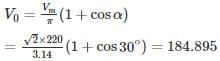The average output current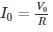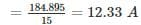*Answer can only contain numeric values
Test: Power Electronics- 4 - Question 7

A single phase full converter is connected to an AC supply of 300 sin 314 t V. It operates at firing angle α = 30° the load current maintained constant at 5A and the load voltage 120 V. Calculate source inductance (in mH).

Detailed Solution for Test: Power Electronics- 4 - Question 7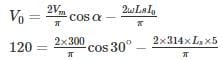120 × 3.14 = 600 cos 30° - 3140 Ls

376.8 = 519.61 – 3140 Ls

-142.82 = -3140 Ls

Ls = 45.48 mH

Test: Power Electronics- 4 - Question 8

A single-phase full bridge diode rectifier is supplied from 220 V, 50 Hz source the load consist of R = 15 Ω and a large Inductance so as to render the load current constant determine RMS value of diode current and supply P.F.

Detailed Solution for Test: Power Electronics- 4 - Question 8

Average value of output voltage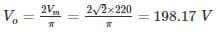Average value of output current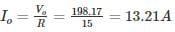R.M.S value of diode current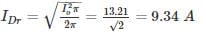Load power = VoIo = 198.17 × 13.21 = 2617.83 W

Input power = VsIs cos ϕ

220 × 13.21 cos ϕ = 2617.83

cos ϕ = 0.9

*Answer can only contain numeric values
Test: Power Electronics- 4 - Question 9

A single phase semiconductor, connected to 220 V 50 Hz Is feeding load R = 15 Ω in series with a large inductance that makes the load current ripple free for firing angle of 60°, calculate the Rectification efficiency ______ in %.

Detailed Solution for Test: Power Electronics- 4 - Question 9

Concept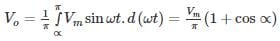R.M.S value of output voltage is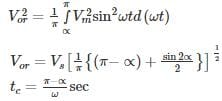Calculation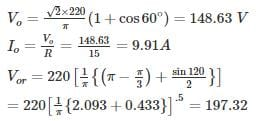The load current is Ripple free

∴ Ior = 197.32/15 = 13.15 A

Pdc = VoIo = 148.63 × 9.91 = 1472.93 W

Pac = VorIor = 197.32 × 13.15 = 2595.46 W

Rectification efficiency =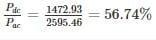*Answer can only contain numeric values
Test: Power Electronics- 4 - Question 10

Find out the ratio of the thyristor to diode RMS current rating in the signal phase half controlled converter for firing angle α = 30°. Assume ripple free contains conduction.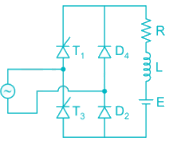Detailed Solution for Test: Power Electronics- 4 - Question 10

Conduction diagram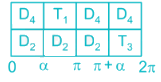From the conduction diagram the thyrsistor conduct for (π – α) radian while the diodes conducts for (π + α) radians. Since the load current is constant.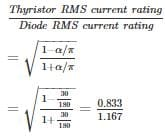= 0.845

## GATE Electrical Engineering (EE) 2023 Mock Test Series

22 docs|274 tests
 Use Code STAYHOME200 and get INR 200 additional OFF Use Coupon Code
Information about Test: Power Electronics- 4 Page
In this test you can find the Exam questions for Test: Power Electronics- 4 solved & explained in the simplest way possible. Besides giving Questions and answers for Test: Power Electronics- 4, EduRev gives you an ample number of Online tests for practice

## GATE Electrical Engineering (EE) 2023 Mock Test Series

22 docs|274 tests